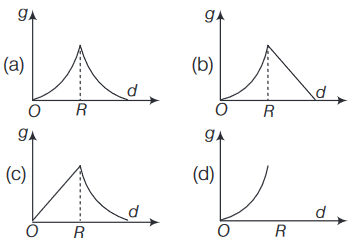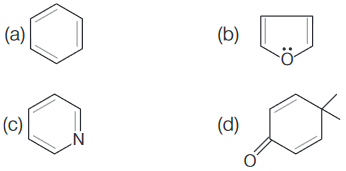### Timezupp!!

-- : --
Are you sure?
Question 1 reset

An observer is moving with half the speed of light towards a stationary microwave source emitting waves at frequency 10 GHz. What is the frequency of the microwave measured by the observer?

(speed of light = ́ 3 X 108 ms-1)

Question 2 reset

In the below circuit, the current in each resistance isQuestion 3 reset

Which one among the following statements is correct?

Question 4 reset

In a common emitter amplifier circuit using an n-p-n transistor, the phase difference between the input and the output voltages will be

Question 5 reset

A capacitance of 2 μF is required in an electrical circuit across a potential difference of 1 kV. A large number of 1 μF capacitors are available which can withstand a potential difference of not more than 300 V. The minimum number of capacitors required to achieve this is

Question 6 reset

A copper ball of mass 100 g is at a temperature T. It is dropped in a copper calorimeter of mass 100 g, filled with 170 g of water at room temperature. Subsequently, the temperature of the system is found to be 75°C. Find the temperature T.

(Given, room temperature = 30°C, specific heat of copper = 0.1 cal/g°C)

Question 7 reset

The variation of acceleration due to gravity g with distance d from centre of the Earth is best represented by

(R = Earth’s radius)Question 8 reset

ΔU is equal to

Question 9 reset
The formation of which of the following polymers involves hydrolysis reaction?
Question 10 reset

The most abundant elements by mass in the body of a healthy human adult are given below:

• Oxygen = 61.4%
• Carbon = 22.9%
• Hydrogen = 10.0%
• Nitrogen = 2.6%

The weight which a 75 kg person would gain if all 1H atoms are replaced by 2H atoms is

Question 11 reset

In the following reactions, ZnO is respectively acting as a/an

• ZnO + Na2O ⟶ NA2ZnO2
• ZnO + CO2 ⟶ ZnCO3
Question 12 reset

3-methyl-pent-2-ene on reaction with HBr in presence of peroxide forms an addition product. The number of possible stereoisomers for the product is

Question 13 reset

1 g of a carbonate (MC2O3) on treatment with excess HCl produces 0.01186 mole of CO2. The molar mass of MC2O3 in g mol-1 is

Question 14 reset

Which of the following molecules is least resonance stabilised?Question 15 reset

If 20 m of wire is available for fencing off a flower-bed in the form of a circular sector, then the maximum area (in sq m) of the flower-bed is

Question 16 reset

If 5(tan2 x - cos2 x) = 2cos 2x + 9,

then the value of cos 4x is

Question 17 reset

If S is the set of distinct values of b for which the following system of linear equations has no solution, then S is

• x + y + z = 1
• z + ay + z = 1
• ax + by + z = 0
Question 18 reset

The statement

(p → q) → [(~ p → q) → q] is

Question 19 resetQuestion 20 reset## Time Over

Please wait while we are submitting your test

Are you sure you want to finish test early?